# FARKAS MIKLÓS ALKALMAZOTT ANALÍZIS SZEMINÁRIUM ELŐADÁSAI 2018/19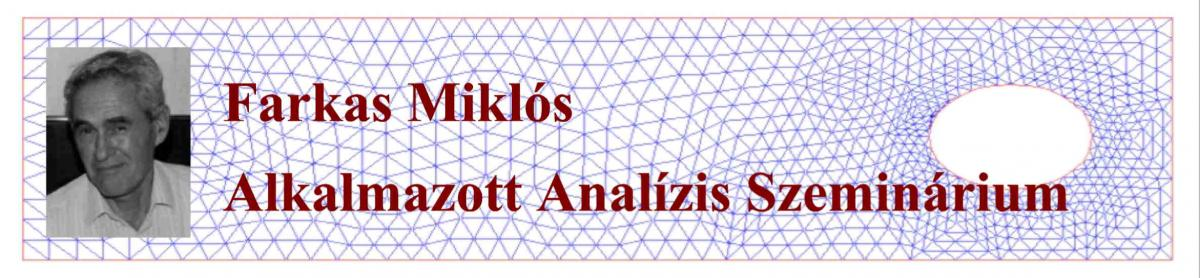9 May 2019Mihály Kovács (Pázmány Péter Catholic University)

Introduction to the semigroup approach for stochastic partial differential equations and their finite element approximation (part 2)

In part 1 of this talk I will give a short introduction to the operator semigroup approach for stochastic partial differential equations driven by Gaussian noise. I will introduce the relevant mathematical background from functional analysis, such as Hilbert-Schmidt and trace-class operators, and from the theory and infinite dimensional stochastic analysis, such as Gaussian measures on Hilbert spaces and stochastic integration in infinite dimensions. To focus on the main issues that arise in this theory, I will only consider equations with additive noise in Hilbert spaces but the concepts introduced can be generalized, with more technical effort, to equations with multiplicative noise and to Banach spaces. I will discuss the stochastic wave equation in more detail as a particular example. In part 2, I will describe a space-time approximation of the linear stochastic wave equation driven by additive nose. For the space-discretization I will introduce the relevant deterministic finite element theory, and for the time-discretization, the relevant theory for rational approximations of the exponential function. Both convergence in the mean-square sense and in the sense of weak convergence of probability measures will be discussed together with sketches of proofs.

2 May 2019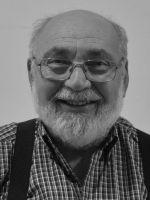Iván Szántó (Federico Santa María Technical University, Valparaiso, Chile)

Cubic System with Limit Cycles and Invariant Algebraic Curves

In this talk, we will show different cubic polynomial systems in the plane, which simultaneously have limit cycles and invariant curves. Examples of Kolmogorov systems and Kukles systems are given. We show the existence of a cubic system having at least one limit cycle bounded by two invariant parabolas. For this system, we will also obtain the necessary conditions for the critical point in the interior of the bounded region to be a center.

25 April 2019Mihály Kovács (Pázmány Péter Catholic University)

Introduction to the semigroup approach for stochastic partial differential equations and their finite element approximation (part 1)

In part 1 of this talk I will give a short introduction to the operator semigroup approach for stochastic partial differential equations driven by Gaussian noise. I will introduce the relevant mathematical background from functional analysis, such as Hilbert-Schmidt and trace-class operators, and from the theory and infinite dimensional stochastic analysis, such as Gaussian measures on Hilbert spaces and stochastic integration in infinite dimensions. To focus on the main issues that arise in this theory, I will only consider equations with additive noise in Hilbert spaces but the concepts introduced can be generalized, with more technical effort, to equations with multiplicative noise and to Banach spaces. I will discuss the stochastic wave equation in more detail as a particular example. In part 2, I will describe a space-time approximation of the linear stochastic wave equation driven by additive nose. For the space-discretization I will introduce the relevant deterministic finite element theory, and for the time-discretization, the relevant theory for rational approximations of the exponential function. Both convergence in the mean-square sense and in the sense of weak convergence of probability measures will be discussed together with sketches of proofs.

11 April 2019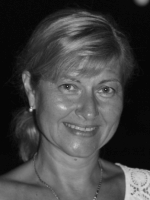Krisztina Kiss (BME, Department of differential equations)

The spread of epidemics in space - as I learned to teach from Miklós Farkas

With this talk, we commemorate Miklós Farkas. It is known that, besides theoretical correctness, he had always emphasized the importance of applications. His lectures on biomathematics were initially targeted mathematical-engineer students who had already been susceptible to applications.

He did not use a projector during his lectures, or give them by memory, but every time he reconsidered his track of thoughts. He had always highlighted the real situation that can be described by the applied model, together with the meaning of the parameters. Mathematical results and formulas were never included without emphasizing the meaning of them. I try to show you why his lectures were popular and engaged the students during his presentation.

Járvány  terjedése térben - ahogyan azt Farkas Miklóstól tanultam tanítani

Ezzel az előadással Farkas Miklósra emlékezünk. Ismert, hogy az elméleti korrektség mellett mindig hangsúlyozta az alkalmazások fontosságát. Biomatematika  előadásait  kezdetben az alkalmazásokra amúgy is fogékony matematikus-mérnök hallgatóknak tartotta.

Előadásait nem vetítette, nem emlékezetből mondta el, hanem minden alkalommal újra átgondolta mondanivalóját. Mindig kiemelte azt a valós szituációt, amely jellemzésére alkalmas a felírt modell, a bennük szereplő paraméterek jelentésével együtt. A matematikai eredmények, formulák sosem szerepeltek a szemléletes jelentés hangsúlyozása nélkül. Azt szeretném most bemutatni, hogy előadásai miért is voltak olyan népszerűek a hallgatóság körében.

4 April 2019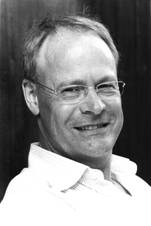Gustaf Söderlind (Lund University, Sweden; guest professor at NUMNETMTA-ELTE reserach group)

Adaptive Linear Multistep Methods - Designing Automatic Step Size Control for Multistep Methods

In a k-step adaptive linear multistep methods the coefficients depend on the k-1 most recent step size ratios. In a similar way, both the actual and the estimated local error will depend on these step ratios. The classical error model has been the asymptotic model, r=chp+1y(p+1)(t)r=chp+1y(p+1)(t), based on the constant step size analysis, where all past step sizes simultaneously go to zero. This does not reflect actual computations with multistep methods, where step size control only affects future steps, not the previous accepted steps. In variable step size implementations, therefore, even in the asymptotic regime, the error model must include the dependence on previous step sizes and step ratios. In this talk we develop dynamic asymptotic models for variable step size computations, and analyze a few new step size controllers accounting for the dynamics in the error model, while keeping the local error near a prescribed tolerance.

28 March 2019Tamás Insperger (BME,  Department of Applied Mechanics)

Human balancing tasks are modelled by differential equations and are compared to experimental observations. First, the classical inverted pendulum model is revisited with respect to stabilizability. Namely, the relation between the reaction time delay and the shortest pendulum length (critical length) of the stick is derived and is demonstrated experimentally. Conclusions are drawn related to human tests, such as stick balancing on the fingertip, balancing a linearly driven inverted pendulum and virtual stick balancing. Second, the ball and beam balancing is considered, where the task is to stabilize a rolling ball at the mid-point of a beam by manipulating the angular position of the beam. Assuming a delayed proportional-derivative feedback mechanism, the governing equation is delay-differential equation. Performance of the control system is analyzed in terms of overshoot and settling time. Experiments over 5-days trials shows that control parameters are tuned to the optimal point associated with minimal overshoot and the shortest settling time. Finally, some further balancing tasks are briefly demonstrated and discussed.

14 March 2019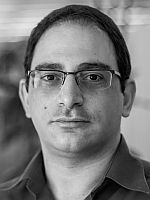Yiannis Hadjimichael (NUMNET MTA-ELTE research group)

Strong-stability-preserving Runge-Kutta methods with downwind-biased operators​

Strong stability preserving (SSP) time integrators have been developed to preserve nonlinear stability properties (e.g., monotonicity, boundedness) of the numerical solution in arbitrary norms or convex functionals, when coupled with suitable spatial discretizations. Currently, all existing general linear methods (including Runge-Kutta and linear multistep methods) either attain small step sizes for nonlinear stability, or they are only first order accurate. In order to obtain larger step sizes discretizations of PDEs that contain both upwind- and downwind-biased operators have been employed.
In this talk, we review SSP Runge-Kutta methods that use upwind- and downwind-biased discretizations in the framework of perturbed Runge-Kutta methods. We show how downwinding improves the SSP properties of time-stepping methods and breaks some order barriers. In particular, we focus on implicit perturbed SSP Runge-Kutta methods that have unbounded SSP coefficient. We present a second- and third-order one-parameter family of perturbed Runge-Kutta methods, for which the CFL-like step-size restriction can be chosen arbitrarily large. The stability of this family of methods is analyzed, and we demonstrate that the desired order of accuracy is obtained for large CFL numbers. Finally, we investigate the computational challenges of the implicit problem and propose ideas that lead to an efficient implementation of Newton's method.

7 March 2019

Evolutionary algorithms

In my talk I'm going to present a quite efficient tool for optimization and constraint satisfaction problems. These methods are called evolutionary or genetic algorithms since their basic concepts mimic the evolution of species. These algorithms were first used to solve discrete problems like scheduling. Later the modified version proved efficient for a class continuous problems, where gradient method and its variants fail either because the objective function isn't differentiable, or the function has many local optima. An example for a specific continuous problem will be presented.

28 February 2019

Multiple solutions of nonlinear partial functional differential equations

We shall consider weak solutions of nonlinear elliptic boundary value problems and initial-boundary value problems for semilinear and nonlinear parabolic differential equations with certain nonlocal terms.  We shall prove theorems on the number of solutions and find multiple solutions. These statements are based on arguments for fixed points of some real functions and operators, respectively, and existence-uniqueness theorems on partial differential equations (without functional terms).

21 February 2019Gustaf Söderlind (Lund University, Sweden; guest professor at NUMNETMTA-ELTE reserach group)

Logarithmic norms and Quadratic forms with applications to Calculus of Variations

The logarithmic norm was introduced in 1958 for matrices, and for the purpose of estimating growth rates in initial value problems. Since then, the concept has been extended to nonlinear maps, differential operators and function spaces. There are applications in operator equations in general, including evolution equations as well as boundary value problems. The logarithmic norm is the extremal value of a quadratic form.

In this talk we outline how logarithmic norms of differential operators can be computed, and how they are related to variational calculus and ellipticity. Thus, while one typically seeks the minimizing function in a variational problem, the logarithmic norm is the corresponding extremal value of the functional associated with a particular symmetrized differential operator. There are also connections to eigenvalue problems for selfadjoint and non-selfadjoint operators. This will also be illustrated with an application to classical singular problems, such as the Bessel equation, as well as to the biharmonic operator.

14 February 2019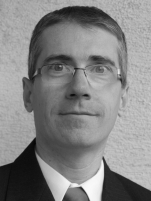Róbert Horváth (BME)

On some qualitative properties of parabolic problems

In this talk we investigate some special qualitative properties of parabolic problems. At the beginning of the talk we review the remarkable qualitative properties of these problems. Then we will turn to two special properties: the first property says that the number of the so-called LL-level points, or specially the number of the zeros, of the solutions must be non-increasing in time. The second property requires a similar property for the number of the local maximizers and minimizers. We show that linear equations and some special nonlinear equations fulfill the above properties in the continuous case. We use the maximum-minimum principles in the proof. Then we generate the numerical solution with the implicit Euler finite difference method and show that the obtained numerical solution satisfies the discrete versions of the above properties without any requirements on the mesh parameters. We show also some numerical test results.

13 December 2018Research reports of PhD students

Teshome Bayleyegn: The method of multiple Richardson extrapolation

Benjámin Borsos: Variable preconditioning for strongly nonlinear elliptic problems

Szilvia György: The stability of systems of linear delay differential equations

Rahele Mosley: Reliable numerical modelling of malaria propagation

Bálint Takács: Epidemic models with spatial dependence

29 November 2018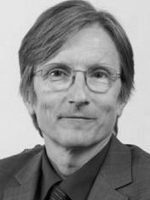Gábor Stépán (BME, Department of Applied Mechanics)

Dynamics of hardware-in-the-loop experiments

A widely used tool of engineering R&D is the hardware-in-the-loop (HIL) experiment. Instead of building the full prototype of a developed machine, only its most critical parts are constructed physically, while the rest of the machine is emulated by means of actuators, sensors, and digital control in between. If the mathematical model of the rest of the machine is available, the control unit can provide a realistic environment for the physically constructed test part. The control, however, introduces digital effects into this system which is originally continuous. From dynamical view-point, the most relevant digital effects are the appearances of delay and zero-order-hold (ZOH). The nonlinear dynamics of the real system and the one emulated by means of the HIL experiment are compared from stability and nonlinear vibrations view-point in case of a brake system where stick-slip phenomenon occurs. The limitations of HIL experiments are identified by means of Hopf bifurcation calculations, numerical simulations and dynamic measurements carried out on the corresponding experimental test rig.

The introductory part of the lecture will present the concept of the ERC advanced grant project and the role of HIL in the development of new milling tools for metal cutting, and the concluding part of the lecture will present the application of the results for the development of HIL experiments in case of high-speed-milling (HSM).

22 November 2018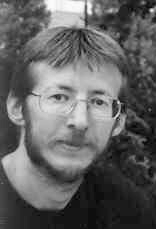János Karátson (ELTE, BME)

Extended finite element methods: a brief introduction

The finite element method (FEM) is a fundamental tool of the numerical solution of real-life problems based on partial differential equations. In the recent decades, various generalizations of the standard FEM have been developed. A lot of such extensions have been motivated by difficulties, arising in physical or engineering problems, that may be cumbersome to overcome with standard FEM techniques. Such situations are the presence of boundary layers, singularities or discontinuities in the solution, complex and/or evolving geometry of the physical domains etc. The tools of extension of the FEM may be enriching the polynomial approximation space with non-polynomial shape functions, allowing general polygonal/polyhedral cells, or use a boundary-unfitted mesh and restricted shape functions (either to a bulk domain or to a surface). This survey type talk gives a brief introduction to the main ideas of some generalized FEMs that use the above ideas: XFEM, VEM, CutFEM and TraceFEM.

15 November 2018

P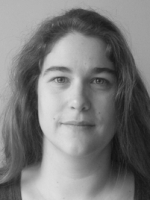etra Csomós (ELTE)

Operator semigroups, innovative integrators, and flood prevention

The talk summarizes our results obtained when studying the possible application of innovative integrators (operator splitting  procedures and exponential integrators) to optimal control problems. We introduce the innovative integrators considered, and present the advantages and drawbacks of their use. After introducing the abstract optimal control problem having a linear quadratic cost function, we prove the convergence of innovative integrators applied to it. To do so, we briefly summarize the main results in operator semigroup theory, used in the proof. As an example, we treat the linear quadratic regulator problem of the one-dimensional and two-dimensional shallow water equations, and illustrate our results by numerical experiments.

The results are based on the joint works with Johannes Winckler (Tübingen) and Hermann Mena (Innsbruck).

• Related papers:​

8 November 2018Barna Garay (Pázmány Péter Chatolic University)

Moving average network examples for asymptotically stable periodic orbits of monotone maps

For a certain type of discrete--time nonlinear consensus dynamics, asymptotically stable periodic orbits are constructed. Based on a simple ordinal pattern assumption, the Frucht graph, two Petersen septets, hypercubes, a technical class of circulant graphs (containing Paley graphs of prime order), and complete graphs are considered -- they are all carrying moving average monotone dynamics admitting asymptotically stable periodic orbits with period 2. Carried by a directed graph with 594 (multiple and multiple loop) edges on 3 vertices, also the existence of asymptotically stable r-periodic orbits, r=3,4,... is shown.

25 October 2018

Mihály Pituk (Departme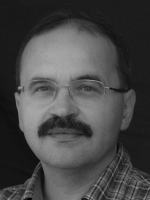nt of Mathematics, University of Pannonia, Veszprém, Hungary)

Ergodicity in nonautonomous linear ordinary differential equations

The weak and strong ergodic properties of the positive solutions of nonautonomous linear ordinary differential equations will be considered. We will show that if the coefficient matrix function is bounded, essentially nonnegative and uniformly irreducible, then the normalized positive solutions are asymptotically equivalent to the Perron vectors of the strongly positive transition matrix at infinity (weak ergodicity). If, in addition, the coefficient matrix function is uniformly continuous, then the convergence of the normalized positive solutions to the same strongly positive limiting vector (strong ergodicity) is equivalent to the convergence of the Perron vectors of the coefficient matrices.

This is a joint work with Professor Christian Pötzsche (Alpen-Adria University Klagenfurt, Austria).

18 October 2018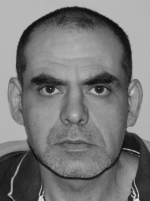Stefan Filipov (Department of Computer Science, Faculty of Chemical System Engineering, University of Chemical Technology and Metallurgy, Sofia, Bulgaria)

Successive application of the linear shooting method for numerical solution of nonlinear two-point boundary value problems

The work to be presented concerns numerical solution of nonlinear two-point boundary value problems. We show that the quasi-linearization method (Newton method on operator level) can be used as a basis to derive (i) the FDM with Newton method and (ii) the shooting by Newton method. The same relation holds for the Picard and the constant-slope methods. Based on these results, we propose (i) a replacement of the FDMs for nonlinear problems (the relaxation methods) by respective successive application of the linear shooting method and (ii) a shooting by Picard method (shooting-projection method). We discuss the advantages of the proposed approaches and present examples.

11 October 2018

Róbert Kovács (BME, Department of Energy Engineering)R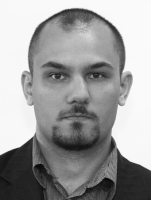Analytical and numerical solutions of dissipative systems​

Our world is not ideal, in reality the processes are dissipative. The framework of non-equilibrium thermodynamics offers lot of possibilities to derive models, constitutive equations that describe the behavior of a dissipative system. The level of modeling is arbitrary, depends on our choice which is reflected by these models. However, they should be remain as simple as possible to be applicable for practical problems in question.

First, the non-equilibrium thermodynamical background and the structure of equations are discussed. Here mostly the parabolic - hyperbolic properties of the resulted partial differential equations (PDE) are emphasized. The role of boundary conditions and their effect on solutions are also presented through different examples. Such example is related to a particular experimental arrangement called heat pulse (or laser flash) experiment that used to detect different dissipative wave propagation phenomena.

In this presentation the way from generating the equations to their solutions for experiments is presented. It covers analytical solution of a PDE for time dependent boundary condition and a particular numerical method that allows us to eliminate certain boundary conditions and related to the specific structure resulted by non-equilibrium thermodynamics.

Numerical solutions: https://www.sciencedirect.com/science/article/pii/S0017931018322002 (arXiv preprint arXiv:1805.01108)

Analytic solutions: https://www.sciencedirect.com/science/article/pii/S001793101831843X (arXiv preprint arXiv:1804.05225)

Theoretical background: https://www.sciencedirect.com/science/article/pii/S0017931014011533

4 October 2018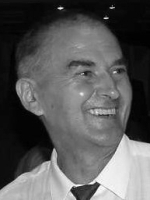Miklós Rontó (University of Miskolc)

New successive approximation method of general non-local boundary value problems

We propose a new successive approximation technique for the solvability analysis and approximate solution of general non-local boundary value  problems for non-linear systems of differential equations with locally Lipschitzian non-linearities. It will be studied the non-linear boundary value problem
dx(t)dt=f(t,x(t)),t∈[a,b],Φ(x)=d,dx(t)dt=f(t,x(t)),t∈[a,b],Φ(x)=d,
where Φ:C([a,b],Rn)→RnΦ:C([a,b],Rn)→Rn is a vector functional (possibly non-linear),  f:[a,b]×Rn→Rn  f:[a,b]×Rn→Rn is a function satisfying the Caratheodory conditions in a certain bounded set DD, which will be concretized later, dd is a given vector and f∈Lip(K,D),f∈Lip(K,D), i.e. ff locally Lipschitzian
|f(t,u)−f(t,v)|≤K|u−v|, for all {u,v}⊂D  a.e.t∈[a,b].|f(t,u)−f(t,v)|≤K|u−v|, for all {u,v}⊂D  a.e.t∈[a,b].
By a solution of the problem, one means an absolutely continuous function satisfying the differential system almost everywhere on [a,b].[a,b]. The analysis is constructive in the sense that it allows one to both study the solvability of the problem and approximately construct its solutions by operating with objects that are determined explicitly in finitely many steps of computation. The practical application of the technique is explained on a numerical example.

27 September 2018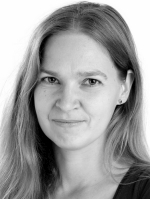Ilona Nagy (BME, Department of Analysis)

Two Limit Cycles in a Two-Species Reaction

Kinetic differential equations, being nonlinear, are capable of producing many kinds of exotic phenomena. However, the existence of multistationarity, oscillation or chaos is usually proved by numerical methods. Here we investigate a relatively simple reaction among two species consisting of five reaction steps, one of the third order. About this reaction we show the following facts (using symbolic methods): the kinetic differential equation of the reaction has two limit cycles surrounding the stationary point of focus type in the positive quadrant. The outer limit cycle is always stable and the inner one is always unstable. We also performed the search for partial integrals of the system and have found one such integral. Application of the methods needs computer help (Wolfram language and Singular) because the symbolic calculations to carry out are too complicated to do by hand. Even to make characteristic drawings is very far from trivial: the inner limit cycle is very small. Some of the methods we use are relatively new, and all of them have never been used in reaction kinetics, although they can be used to have similar exact results on other models.

20 September 2018

Professors Gustaf Söderlind and Carmen Arévalo will present three talks during their visit to Budapest. All three talks deal with adaptive methods of ODEs. Professor Söderlind’s first talk will be held at the Seminar on Applied Analysis of the Department of Applied Analysis at ELTE university (details below), while his second talk together with Professor Arévalo’s talk will be presented at the Miklós Farkas Seminar. Although professor Söderlind’s talks form a complete presentation together, yet they can be followed separately.Gustaf Söderlind (Lund University, Sweden)

Adaptive Time-Stepping. Part II. Time transformations applied to reversible Hamiltonian dynamics and weakly dissipative systems

In the second talk on time step adaptivity, we focus on the special needs of conservative dynamical systems. This includes Hamiltonian problems, and weakly dissipative systems. In integrable Hamiltonian problems, the mathematical solution is time reversible, which precludes the use of classical controllers, which adapt the step size to manage the error observed in previous steps. Instead, a time reversible tracking algorithm is developed, which allows full reversibility of the adaptive computational process. This is shown to preserve first integrals over long times, and even improves the accuracy over constant step size symplectic integrators. We demonstrate the procedure in two examples from celestial mechanics, and then proceed to demonstrate how a similar approach can be combined with splitting methods in weakly dissipative systems. The latter approach has been put to effective use in rolling bearing dynamic simulation.

The first part of the talk:

Adaptive Time-Stepping. Part I. How control theory and digital filters enhance the performance of IVP solvers

Seminar on Applied Analysis, 17 September 2018, 14:00, Department of Applied Analysis and Computational Mathematics, ELTE, Pázmány P.  1/C, room 3.719

In two talks we will describe a systematic approach to time-stepping adaptivity in the numerical integration of initial value problems. The first part will deal with the proper construction of discrete controllers that keep the local error equidistributed along the solution trajectory. The controllers will be based both on classical constructions such as proportional-integral controllers, and on modern digital low-pass filters, which are designed to maintain a smooth step size sequence. We will demonstrate that a regular step size sequence is of benefit as it increases the computational stability — small changes in the error tolerance will only produce a small change in the accuracy of the computed solution. While computational speedup is often only modest, the quality of the computed solution as well as the reliability of the software can sometimes be remarkably improved. Presentation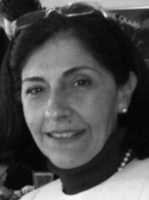Carmen Arévalo (Lund University, Sweden)

An intrinsically adaptive formulation of multistep methods

Multistep methods are important tools for solving ordinary differential equations with initial conditions. In order for these methods to be efficient they must be adaptive, that is, they must allow the choice of an appropriate step-size for each integration step. We present a comprehensive way of formulating multistep methods that is adaptive by construction and show how this methodology can be applied to particular situations. We also show the application to strong stability preserving methods, used to solve ODEs arising from the semi-discretization of time-dependent partial differential equations (PDEs), especially hyperbolic PDEs with shocks. We finally demonstrate how we apply the formulation to differential algebraic equations (DAEs), an ODE system coupled with algebraic constraints.